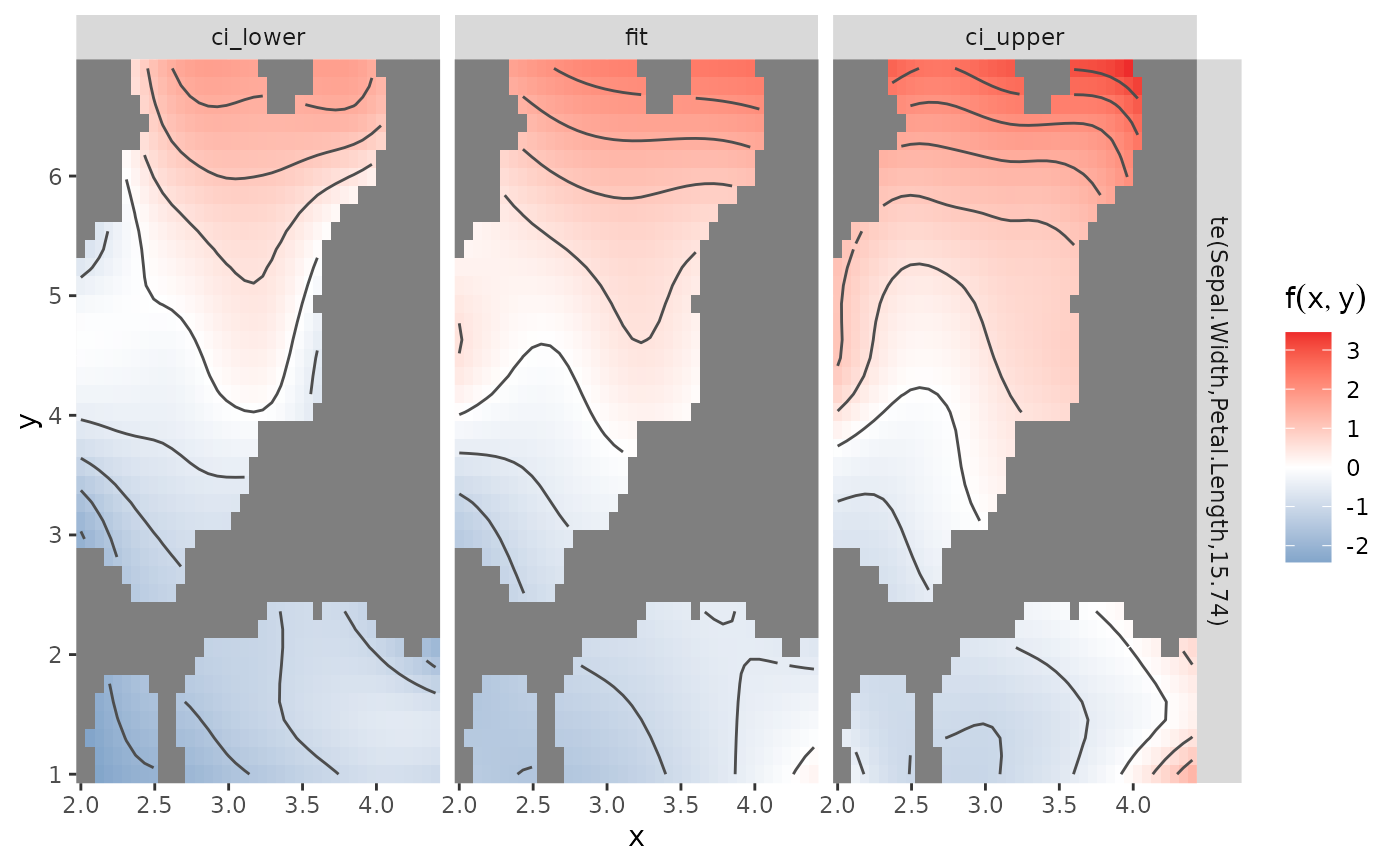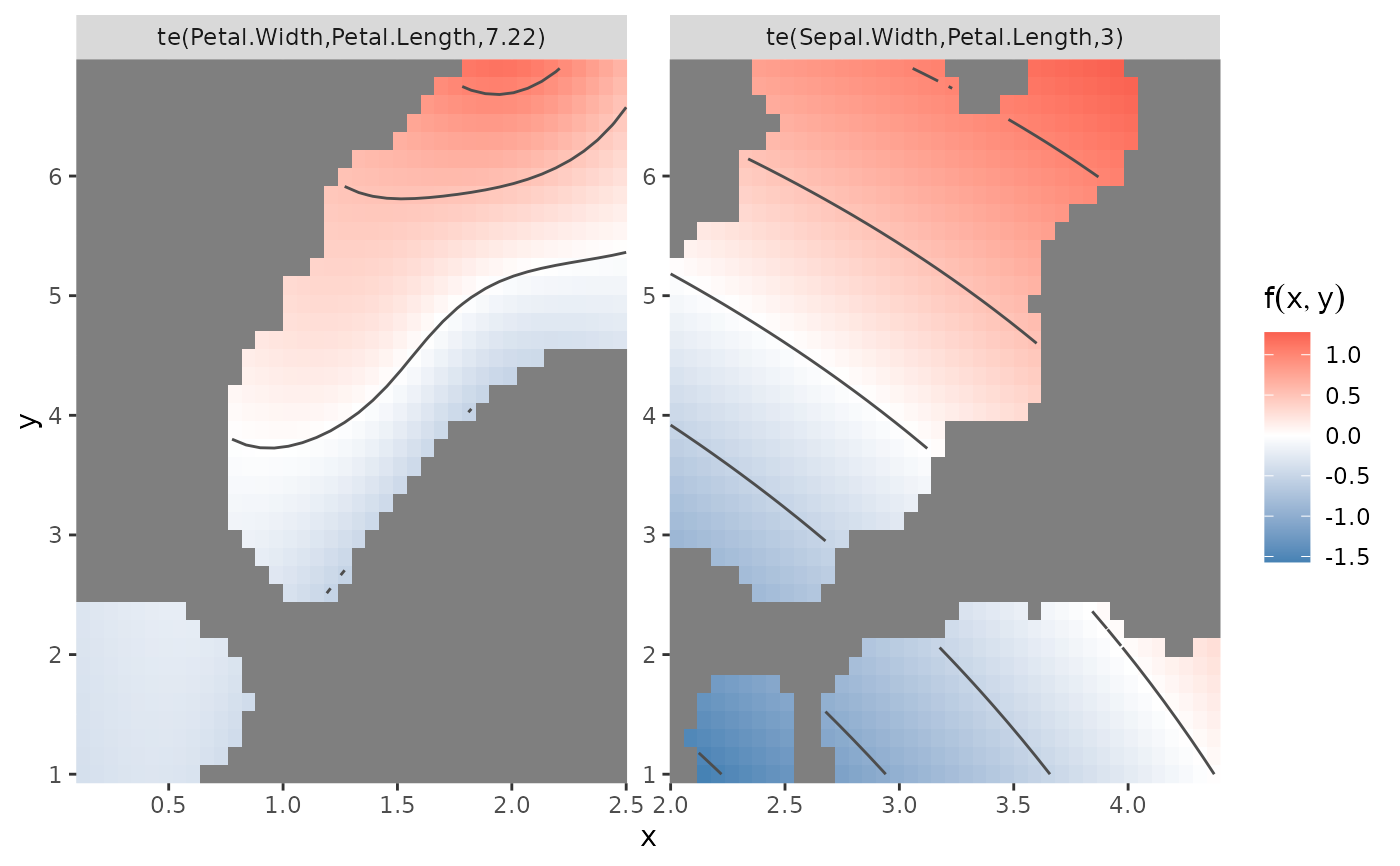Given a gam model this convenience function returns a ggplot2 object depicting 2d smooth terms specified in the model as heat/contour plots. If more than one 2d smooth term is present individual terms are faceted.

gg_tensor(x, ci = FALSE, ...)

## Arguments

x

a fitted gam object as produced by gam().

ci

A logical value indicating whether confidence intervals should be calculated and returned. Defaults to TRUE.

...

Further arguments passed to plot.gam

tidy_smooth2d

## Examples

g <- mgcv::gam(Sepal.Length ~ te(Sepal.Width, Petal.Length), data=iris)
gg_tensor(g)
#> Warning: Removed 647 rows containing non-finite values (stat_contour()).gg_tensor(g, ci=TRUE)
#> Warning: Removed 1941 rows containing non-finite values (stat_contour()).gg_tensor(update(g, .~. + te(Petal.Width, Petal.Length)))
#> Warning: Removed 1624 rows containing non-finite values (stat_contour()).
#> Warning: Raster pixels are placed at uneven horizontal intervals and will be shifted
#> ℹ Consider using geom_tile() instead.